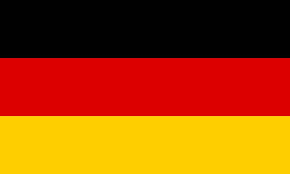# Distance Point-PlaneEnter a point and a plane. Mathepower calculates the distance between the point and the plane.

P(||)

Choose how the plane is given.
• parametric equation:

 E: x =+ r+ s• Coordinate form:

• Point-normal form:

 E: (x-)⋅=0
• Given through three points